Next: SMOOTHING WITH BOX AND Up: LEAKY INTEGRATION Previous: Plots

## Two poles

Integration twice is an operation with two poles. Specifically,(9)
Notice that the signal is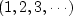,which is a discrete representation of the function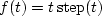.Figure 2 shows the result when the two integrations are leaky integrations.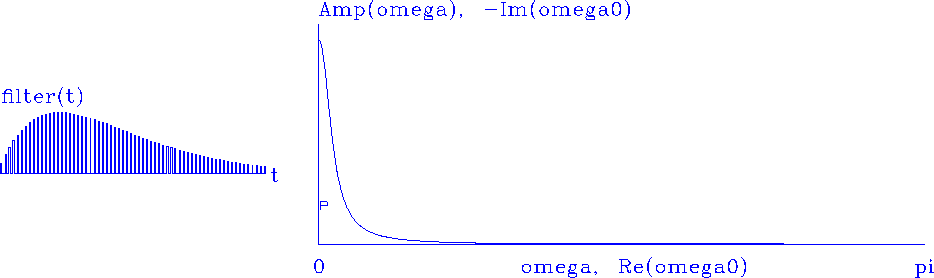leak2
Figure 2
A cascade of two leaky integrators.

We see the signal begin as t but then drop off under some weight that looks exponential. A second time-derivative filterhas an amplitude spectrum.Likewise, a second integration has an amplitude spectrum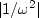,which is about what we see in Figure 2, except that atleaky integration has rounded off the.

Instead of allowing two poles to sit on top of each other (which would look like just one pole), I moved the pole slightly offso that.As in Figure, another pole is included (but not shown) at negative frequency. This extra pole is required to keep the signal real. Of course the two poles are very close to each other. The reason I chose to split them this way is to prepare you for filters where the poles are far apart.

## EXERCISES:

1. Show that multiplication by (1 - Z) in discretized time is analogous to time differentiation in continuous time. Show that dividing by (1 - Z) is analogous to integration. What are the limits on the integral?
2. A simple feedback operation is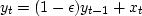.Give a closed-form expression for the output yt if xt is an impulse. Estimate the decay timeof your solution (the time it takes for yt to drop to e-1y0)? For small,say = 0.1, .001, or 0.0001, what is?
3. Find an analytic expression for the plot on the right side of Figure 1 as a function of.Show that it is like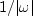.
4. In continuous time, the signal analogous to that in Figure 2 is te-t. What is the analogous frequency function?Next: SMOOTHING WITH BOX AND Up: LEAKY INTEGRATION Previous: Plots
Stanford Exploration Project
10/21/1998